# Compare Strings in Java

Wikitechy | 3507 Views | java | 13 Jun 2016

• In java we can compare the string in three ways:

1. By equals() method

2. By = = operator

3. By compareTo() method

#### Equals Method:

• Equals() method compare two Strings for content equality.
• So if two string contains same letters, in same order and in same case they will be equals by equals() method.
• Equals() method is defined in Object class and String class that overrides character based comparison.
• This method returns true when two String objects hold the same content (i.e. The same values).

#### For Example: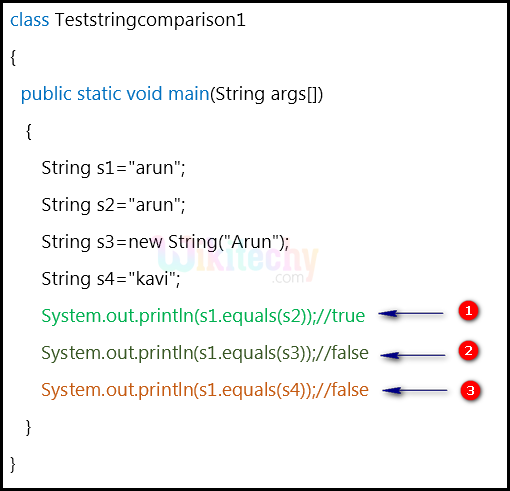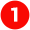Here in this line we compare the strings “s1” and “s2” in which both are equal. (So, it returns “true”)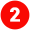Similarly, in this line we compare the strings “s1” and “s3” in which both are not equal because “s1” variable contains the string “arun” in lower case but “s2” variable contains the string “Arun” in which the first letter in upper case. (So it as returns “false”)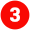In this line we compare the strings “s1” and “s4” in which both are not equal because “s1” variable contains the string “arun” and “s2” variable contains the string “kavi” So it as returns “false”.

#### == Operator:

• == operator is used to compare two objects in Java.
• == is an operator and equals() is the method.
• But == operator compares reference / memory location of objects in the heap, whether they point to the same location or not.
• whenever we create any object using the operator new it will create new memory location for that object.
• So we use == operator to check memory location or address of two objects whether its same or not.

#### For Example: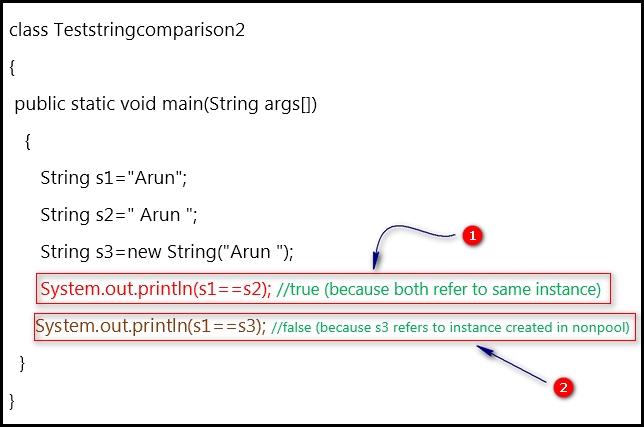In this line we compare the strings “s1” and “s2” in which both are equal because “s1” variable contains the string “Arun” and “s2” variable contains the string “Arun”. So it as returns “false”.Similarly, in this line we compare the strings “s1” and “s3” and it returns “false”. Because “s1” is a variable whereas  “s3” is an  object

#### compareTo() method:

• The String compareTo() method compares values lexicographically and returns an integer value that describes if the first string is less than, equals to or greater than the second string.
• Suppose s1 and s2 are two string variables.
• s1 == s2 : returns “0”
• s1 > s2   : returns “positive value”
• s1 < s2   : returns “negative value”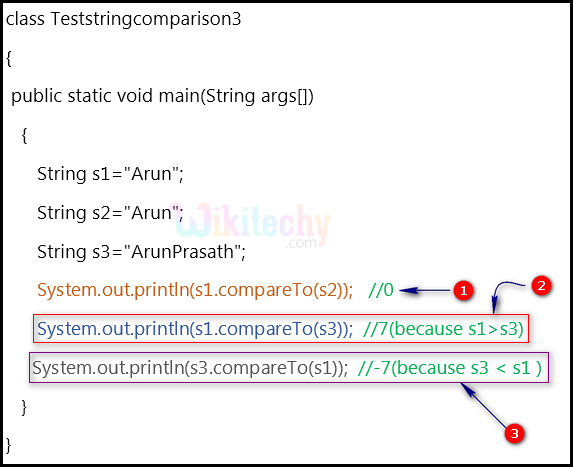Here in this line we compare the strings “s1” and “s2” in which both are equal. (So, it returns “0”)In this line we compare the strings “s1” and “s3” in which both are not equal because “s1” variable contains the string “Arun” & “s3” variable contains the string “ArunPrasath” comparatively s1>s3 So it as returns the maximum difference character value as “7”.Similarly, we compare the strings “s3” and “s1” in which both are not equal because “s1” variable contains the string “ArunPrasath” & “s3” variable contains the string “Arun” comparatively s1<s3 So it as returns the minimum difference character value “-7”.

• J2SE 1.3
• J2SE 1.4
• J2SE 5.0
• Java SE 6
• Java SE 7
• Java SE 8

#### Related Tags:

• How do I compare strings in Java?
• String Comparison in Java
• Java Examples - Comparing two strings
• equals(), compareTo(), and compare()
• Comparing Strings and Portions of Strings
• Java program to compare two strings
• How to Compare two String in Java
• Java string compare sample code examples
• Java String Comparison

Bug Bounty
Webinar